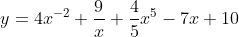## The Derivative

Find the derivative of:Hint
Rewriting the equation:
$$y=4x^{-2}+9x^{-1}+\frac{4}{5}x^5-7x^1+10x^0$$$Hint 2 The power rule for the first derivative: $$\frac{d}{dx}[x^n]=n\cdot x^{n-1}$$$
The power rule for the first derivative:
$$\frac{d}{dx}[x^n]=n\cdot x^{n-1}$$$Rewriting the problem statement’s equation to be in the power rule’s format: $$y=4x^{-2}+9x^{-1}+\frac{4}{5}x^5-7x^1+10x^0$$$
Taking the first derivative via power rule:
$$y'=(-2)4x^{-2-1}+(-1)9x^{-1-1}+(5)\frac{4}{5}x^{5-1}-(1)7x^{1-1}+(0)10x^{0-1}$$$$$y'=-8x^{-3}+-9x^{-2}+4x^{4}-7x^{0}+0$$$
$$y'=-8x^{-3}+-9x^{-2}+4x^{4}-7$$$$$y'=-8x^{-3}+-9x^{-2}+4x^{4}-7$$$